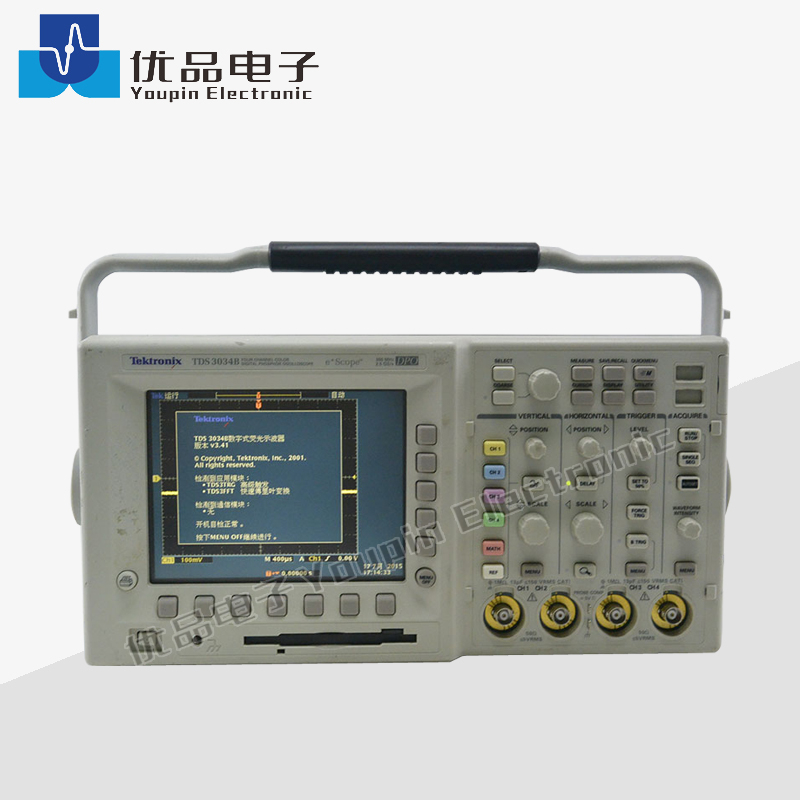Sie sind hier: » » » » Tektronix TDS3034B Digital Phosphor OscilloscopeAnteil an:

# Tektronix TDS3034B Digital Phosphor Oscilloscope

Tektronix TDS3034B Digital Phosphor Oscilloscope: 4 Channels, 300 MHz, 2.5 GS/s
• Tektronix

• TDS3034BTektronix TDS3034B OSCILLOSCOPE The Tektronix TDS3034B oscilloscope is a graph-displaying device – it draws a graph of an electrical signal. In most applications, the graph shows how signals change over time: the vertical (Y) axis represents voltage and the horizontal (X) axis represents time. The intensity or brightness of the display is sometimes called the Z axis. The Tektronix TDS3034B oscilloscope's simple graph can tell you many things about a signal, such as: the time and voltage values of a signal, the frequency of an oscillating signal, the “moving parts” of a circuit represented by the signal, the frequency with which a particular portion of the signal is occurring relative to, other portions, whether or not a malfunctioning component is distorting the signal, how much of a signal is direct current (DC) or alternating current (AC) and how much of the signal is noise and whether the noise is changing with time.Tektronix TDS3034B OSCILLOSCOPE The Tektronix TDS3034B oscilloscope is a graph-displaying device – it draws a graph of an electrical signal. In most applications, the graph shows how signals change over time: the vertical (Y) axis represents voltage and the horizontal (X) axis represents time. The intensity or brightness of the display is sometimes called the Z axis. The Tektronix TDS3034B oscilloscope's simple graph can tell you many things about a signal, such as: the time and voltage values of a signal, the frequency of an oscillating signal, the “moving parts” of a circuit represented by the signal, the frequency with which a particular portion of the signal is occurring relative to, other portions, whether or not a malfunctioning component is distorting the signal, how much of a signal is direct current (DC) or alternating current (AC) and how much of the signal is noise and whether the noise is changing with time.